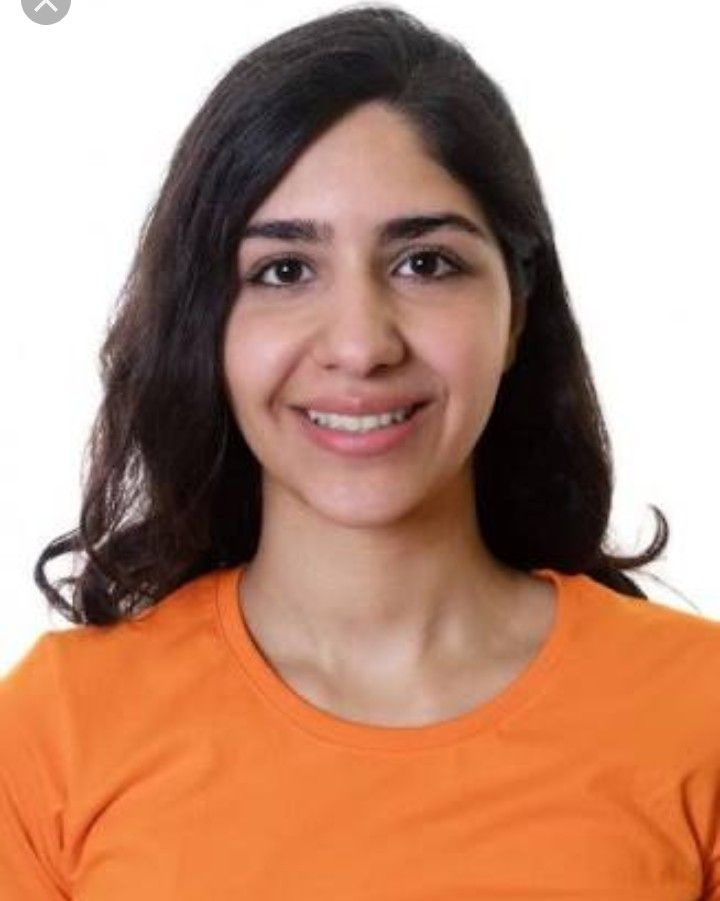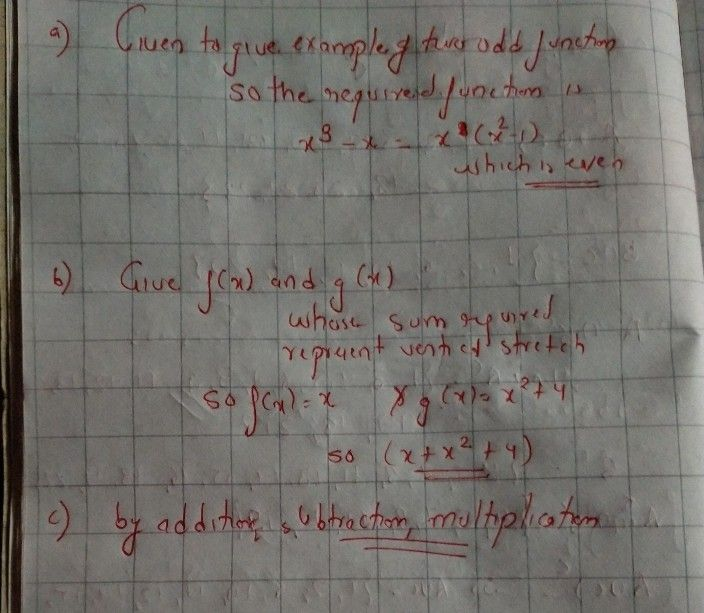Symbol
Problem$a\right)$ $Gi$ Give an example of two odd functions $f\left(x\right)$ and $g\left(x\right)$ wvhose difference is $an$ even function. $b\right)$ Give an example of two functions $f\left(x\right)$ and $g\left(x\right)$ whose sum represent $a$ vertical stretch apPplied to one of the functions. Explain the three ways $y0y$ $C81$ determine the composition of two functions, $f$ and $g7$
Other
Search count: 109
SolutionQanda teacher - seena215Student
Thank you very much
Can i have questionQanda teacher - seena215
Dear, If you have any doubt feel free to ask:) Please evaluate(rate the answer):- 1. Click on top right corner EVALUATE button. 2. Select 5 star. 3. Click on gift coins + three times(C3000)(Not reduce your coins). 4. Click on DONE button on top right corner.
you can ask seperatley as in one chat room.one doubt is allowedStudent
F(x) multiply g(x) in B right?Qanda teacher - seena215
yesStudent
Thank you vey much